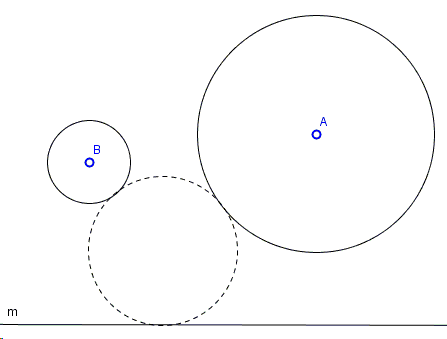# LCC: Apollonius' Problem with Two Circles and a Line

Below I give an elementary Euclidean construction to a variant of the Problem of Apollonius:

Find a circle tangent to two given circles $(A)$ and $(B)$ and a given line $m$:(The applet below illustrates the construction.)

Solution### Construction

Find a circle tangent to two given circles $(A)$ and $(B)$ and a given line $m$:Assume that radii of circles $(A)$ and $(B)$ are $a$ and $b$, respectively, and that $b\lt a.$Form circle $(A,a-b)$ with center $A$ and radius $a-b.$ Also, shift line $m$ away from the two circles to position $m_b$, at distance $b$ from $m.$ We may now may solve the PLC problem with point $B$, line $m_b$, and circle $(A,a-b)$. If that problem has a solution $(X,r)$, then $(X, r-b)$ solves the original problem.

Since the PLC problem has four solution and line $m$ can be moved in either of the two directions, the problem may have up to 8 solutions.

### References

1. N. Altshiller-Court, College Geometry, Dover, 1980, #515